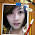## 2013/08/21

### 全球僅2%人破解的摩斯密碼？

5+3+2=151022 摩斯密碼全球僅2%人破解(蘋果日報)

5+3+2=151022
15=5+10 (第一個數加10)
10=3+  7 (第二個數加  7)
22=2+20 (第三個數加20)

5+3+2=151022
15=3*5   (第二個數乘以在他後一位的質數np1)
10=5*2   (第一個數乘以在他前兩位的質數pp2)
22=2*11 (第三個數乘以在他後四位的質數np4)

5+3+2=151022
15=15
10=10
22=22

#### 6 則留言:

1.同意，這比較像猜謎更甚於數學
不過這道題流傳的時候，題幹有四組式子讓人去找數字規律，所以算還好啦

2.3+4+9=12273636
5+6+7=30354251
7+2+5=14351026
9+8+3=

3.72272445

4.請問45是怎麼解的？

5.7*2+7*5-2=45

6.7*2+7*5-2=45??
式子是否有誤?